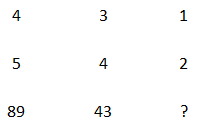Question 18

# Select the missing number from the given options.Solution

4, 5, 89 pattern is $$\left(4\times\ 5\right)\times\ 4+\left(4+5\right)=89$$

3, 4, 43 pattern is $$\left(3\times\ 4\right)\times\ 3+\left(3+4\right)=43$$

1, 2, 5 pattern is $$\left(1\times\ 2\right)\times\ 1+\left(1+2\right)=5$$

• Free SSC Study Material - 18000 Questions
• 230+ SSC previous papers with solutions PDF